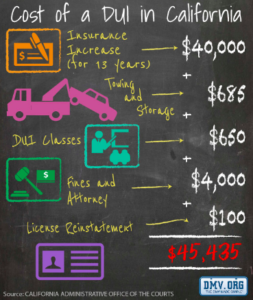## Putting it Together: Linear Inequalities

At the start of this module, we continued the story of Joan who had been drinking at a party. She was ready to drive home when we posed a question:

• Given any amount that she drinks, can you figure out how much time should pass before she can drive safely and legally?

Previously, we used the same formula commonly used by forensic scientists to calculate blood alcohol content to find her blood alcohol content to determine whether she was within the legal limit to drive:

$B=-0.015t +\left(\dfrac{2.84N}{Wg}\normalsize\right)$

where

• B = percentage of BAC
• t = number of hours since the first drink
• N = number of “standard drinks” (a standard drink is one $12$-ounce beer, one $5$-ounce glass of wine, or one $1.5$-ounce shot of liquor). N should be at least $1$.
• W = weight in pounds
• g = gender constant: $0.68$ for men and $0.55$ for women

We calculated Joan’s BAC as $0.092\%$ which meant that she’s probably experiencing blunted feelings, reduced sensitivity to pain, euphoria, disinhibition, and extroversion. In addition, her reasoning, depth perception, peripheral vision, and glare recovery are probably impaired.

We also found that if the legal limit were $0.08\%$, she would not be within the legal limit to drive.Cost of a DUI in California.

Now that we know about the algebra of inequalities, we can answer the new question about Joan.

• Given any amount that she drinks, can you figure out how much time should pass before she can drive safely and legally?

This question takes a little more thinking. Let’s continue with our example of three standard drinks to answer it. In the first question, we solved for BAC given a specific number of drinks, amount of time, and Joan’s weight. What is different about this question? Are we solving an equation or an inequality?

Let’s start with what we know. We want to know how long it will take to be within the legal limit to drive, and we know that $1.5$ hours is not enough. The word within is often associated with inequalities. We can translate this as “When will this person’s BAC be less than $0.08\%$?”

When writing an inequality based on a situation, one of the trickiest parts is knowing which direction the inequality sign will go. In other words, which of the following inequalities will answer our question correctly?

$\text{0.08% }\lt -0.015t +\left(\dfrac{2.84N}{Wg}\normalsize\right)$

or

$\text{0.08% }\gt -0.015t +\left(\dfrac{2.84N}{Wg}\normalsize\right)$

Using words to translate what these expressions mean is often helpful:

$\text{0.08% }\lt -0.015t +\left(\dfrac{2.84N}{Wg}\normalsize\right)$

Translation: For a limit of $0.08\%$, what time will make this side greater than $0.08\%$?

That doesn’t seem right. We want to know how much time will make the right-hand side less than $0.08\%$, so we would want to choose the other one:

$\text{0.08% }\gt -0.015t +\left(\dfrac{2.84N}{Wg}\normalsize\right)$

Solving for time gives us the following:

$\begin{array}{c}\text{0.08 }\gt -0.015t +0.1147\\{-0.0347}\gt -0.015t\end{array}$

Divide both sides by $-0.015$ and remember to change the direction of the inequality sign. That gives us the following:

$2.31<t$

We have our answer to this new question. According to the expression above, our answer is “any time greater than $2.3$ hours will be long enough for Joan to be within the legal limit for driving after she’s consumed three standard drinks.” We hope Joan takes our advice and gets home okay.

Progressive Effects of Alcohol
BAC (% by vol.) Behavior Impairment
$0.001–0.029$
• Average individual appears normal
• Subtle effects that can be detected with special tests
$0.030–0.059$
• Mild euphoria
• Relaxation
• Joyousness
• Talkativeness
• Decreased inhibition
• Concentration
$0.060–0.099$
• Blunted feelings
• Reduced sensitivity to pain
• Euphoria
• Disinhibition
• Extroversion
• Reasoning
• Depth perception
• Peripheral vision
• Glare recovery
$0.100–0.199$
• Over-expression
• Boisterousness
• Possibility of nausea and vomiting
• Reflexes
• Reaction time
• Gross motor control
• Staggering
• Slurred speech
• Temporary erectile dysfunction
$0.200–0.299$
• Nausea
• Vomiting
• Emotional swings
• Partial loss of understanding
• Impaired sensations
• Decreased libido
• Possibility of stupor
• Severe motor impairment
• Loss of consciousness
• Memory blackout
$0.300–0.399$
• Stupor
• Central nervous system depression
• Loss of understanding
• Lapses in and out of consciousness
• Low possibility of death
• Breathing
• Dysequilibrium
• Heart rate
$0.400–0.500$
• Severe central nervous system depression
• Coma
• Possibility of death
• Breathing
• Heart rate
• Positional Alcohol Nystagmus
$>0.50$
• High risk of poisoning
• High possibility of death
• Life